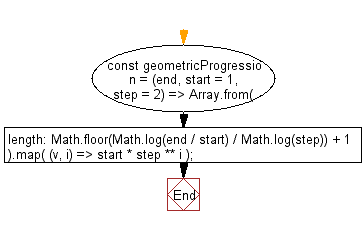# JavaScript: Initializes an array containing the numbers in the specified range

## JavaScript fundamental (ES6 Syntax): Exercise-222 with Solution

Write a JavaScript program that initializes an array containing the numbers in the specified range where start and end are inclusive and the ratio between two terms is step. Returns an error if step equals 1.

• Use Array.from(), Math.log() and Math.floor() to create an array of the desired length, Array.prototype.map() to fill with the desired values in a range.
• Omit the second argument, start, to use a default value of 1.
• Omit the third argument, step, to use a default value of 2.

Sample Solution:

JavaScript Code:

``````//#Source https://bit.ly/2neWfJ2
const geometricProgression = (end, start = 1, step = 2) =>
Array.from({ length: Math.floor(Math.log(end / start) / Math.log(step)) + 1 }).map(
(v, i) => start * step ** i
);
console.log(geometricProgression(256));
console.log(geometricProgression(256, 3));
console.log(geometricProgression(256, 1, 4));
```
```

Sample Output:

```[1,2,4,8,16,32,64,128,256]
[3,6,12,24,48,96,192]
[1,4,16,64,256]
```

Pictorial Presentation:Flowchart:Live Demo:

See the Pen javascript-basic-exercise-222-1 by w3resource (@w3resource) on CodePen.

Improve this sample solution and post your code through Disqus

What is the difficulty level of this exercise?

Test your Programming skills with w3resource's quiz.

﻿

## JavaScript: Tips of the Day

How to check whether a string contains a substring in JavaScript?

ECMAScript 6 introduced String.prototype.includes:

```const string = "foo";
const substring = "oo";

console.log(string.includes(substring));
```

includes doesn't have Internet Explorer support, though. In ECMAScript 5 or older environments, use String.prototype.indexOf, which returns -1 when a substring cannot be found:

```var string = "foo";
var substring = "oo";

console.log(string.indexOf(substring) !== -1);
```

Ref: https://bit.ly/3fFFgZv